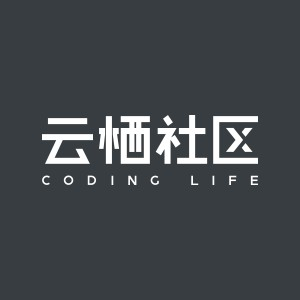《Two Dozen Short Lessons in Haskell》学习（十一）- Tuples

+关注继续查看

第十一章 元组

1 The type of the tuple ("X Windows System", 11, "GUI") is

a (String, Integer, Char)

b (String, Integer, String)

c (X Windows System, Eleven, GUI)

d (Integer, String, Bool)

2 After the following definition, the variables x and y are, respectively,

HASKELL DEFINITION • (x, y) = (24, "XXIV")

a both of type Integer

b both of type String

c an Integer and a String

d undefined — can’t define two variables at once

3 After the following definition, the variable x is

HASKELL DEFINITION • x = (True, True, "2")

a twice True

b a tuple with two components and a spare, if needed

c a tuple with three components

d undefined — can’t define a variable to have more than one value

4 After the following definition, the variable x is

HASKELL DEFINITION • x = 16 ‘divMod‘ 12

a 1 +4

b 16÷4

c 1 × 12 + 4

d (1, 4)

5 The formula divMod x 12 == x ‘divMod‘ 12 is

a (x ‘div‘ 12, x ‘mod‘ 12)

b (True, True)

c True if x is not zero

d True, no matter what Integer x is

6 In a definition of a tuple

a both components must be integers

b the tuple being defined and its definition must have the same number of components

c surplus components on either side of the equation are ignored

d all of the above

=========================================================

=========================================================

1 b

2 c

3 c

4 d

divMod是个函数，通常的写法把参数跟在函数名的后面，而divMod就可以把函数名放在二个参数中间。

divMod返回一个元组，元组的第一个元素是商（取整后），第二个元素是余数。

5 d

6 b

(2,3)与(2,3,4)是两种不同的元组，前者的类型是(Integer, Integer)，后者的类型是(Integer, Integer, Integer)，这两个元组是不能相互比较的。

http://www.cnblogs.com/speeding/1193 0less学习-带参数混合

740 02416 0less 学习-变量

684 0less学习-混合

610 01163 010493 0Swift 学习之Using Swift mix and match, network: 写rss阅读器
1164 01195 0
2010

0

《2021云上架构与运维峰会演讲合集》

《零基础CSS入门教程》

《零基础HTML入门教程》